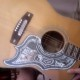### it won’t do you a bit of good to review math

Consider a set of five objects.

In fact, consider the “canonical” set of five objects: {0, 1, 2, 3, 4}.

There are then ten ways to form pairs of these objects, as one sees from the following display:
04 03 02 01
14 13 12
24 23
34
.

(Note that by “pairs”, I refer to unordered pairs. For example, “40” doesn’t occur in the display because this represents the same pair of objects as “04”. In fact, since {4, 0} = {0, 4} as sets, one could formalize the notion of “pairs” as I’m using it here by referring to sets-of-two-objects from our original five-set. Indeed, I’d do it this way despite the extra typing if I wasn’t afraid it would then look harder to read.)

Now let’s call the objects of this ten-set
{01, 02, 03, 04, 12, 13, 14, 23, 24, 34}
the points of a “space” called D (for Desargues). “D” itself is then this set of points, together with a collection of certain (unordered) triples of points. The triples themselves are called the lines of D.

Here are the lines of D.
{01, 02, 12}
{01, 03, 13}
{01, 04, 14}
{02, 03, 23}
{02, 04, 24}
{03, 04, 34}
{12, 13, 23}
{12, 14, 24}
{13, 14, 34}
{23, 24, 34}
.

To form a line, take any three objects from {0, 1, 2, 3, 4} and form all three possible pairs.

One now has a geometry-free construction of the “space” I’ve been examining here in the blog. In particular, each point appears in exactly three lines (just as each line contains exactly three points).

I intended more of this today. But, to my delighted surprise, duty calls: I’m meeting a new tutee in a couple hours and have to go catch the bus.

1.vlorbik

http://www.azimuthproject.org/azimuth/show/Experiments+in+Markov+chains

desargues graph in chemistry.
spotted at _this_week’s_finds_.

2.vlorbik

i’m not at all sure this /*
One now has a geometry-free construction of the “space” I’ve been examining here in the blog.*/
is true. it will require more fiddling
for me to become convinced either way.

meanwhile, here’s some geometry-ed:
http://en.wikipedia.org/wiki/Van_Hiele_model
.

• ## (Partial) Contents Page

Vlorbik On Math Ed ('07—'09)
(a good place to start!)